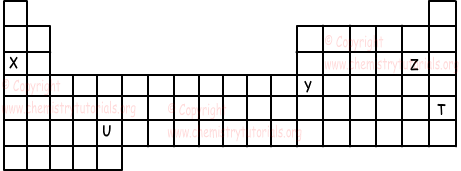EN | ES | DE | NL | RU

## Finding Location of Elements in Periodic Table with Examples

Finding Location of Elements in Periodic Table with Examples

a) Finding Period of Elements:

Period of the element is equal to highest energy level of electrons or principal quantum number. Look at following examples for better understanding;

16S: 1s22s22p63s23p4 3 is the highest energy level of electrons or principal quantum number. Thus period of S is 3.

23Cr: 1s22s22p63s23p64s23d4 4 is the highest energy level of electrons or principal quantum number. Thus period of Cr is 4.

b) Finding Group of Elements:

Group of element is equal to number of valence electrons of element or number of electrons in the highest energy level of elements. Another way of finding group of element is looking at sub shells. If last sub shell of electron configuration is "s" or "p", then group becomes A.

19K: 1s22s22p63s23p64s1 Since last sub shell is "s" group of K is A.

35Br: 1s22s22p63s23p64s23d104p5 Since last sub shell is "p" group of Br is A.

Elements in group B have electron configuration ns and (n-1)d, total number of electrons in these orbitals gives us group of element. Look at following examples.

26Fe: 1s22s22p63s23p64s23d6 6+2=8 B group

Here are some clues for you to find group number of elements.

Last Orbital Group

ns1 1A

ns2 2A

ns2np1 3A

ns2np2 4A

ns2np3 5A

ns2np4 6A

ns2np5 7A

ns2np6 8A

Last Orbital Group

ns2(n-1)d1 3B

ns2(n-1)d2 4B

ns2(n-1)d3 5B

ns2(n-1)d4 or ns1(n-1)d5 6B

ns2(n-1)d5 7B

ns2(n-1)d6 8B

ns2(n-1)d7 8B

ns2(n-1)d8 8B

ns2(n-1)d9 or ns1(n-1)d10 1B

ns2(n-1)d10 2B

Example: Find period and group of 16X.

16X: 1s22s22p63s23p4

3. period and 2+4=6 A group

Example: Find period and group of 24X.

24X:1s22s22p63s23p64s23d4

4. period and 4+2=6 B group

Groups and Periods of elements are found according to their neutral states. Ions and isotopes of elements are not shown in periodic table.

Example: If X+2 ion has 10 electrons, find its group and period number.

Number of protons=10 + 2=12

In neutral element, number of proton is equal to number of electrons. Thus, X has 12 electrons in neutral state. We write electron configuration according to neutral state of element.

12X=1s22s22p63s2

Period number is 3

Group number is 2 and group is A(last orbital is "s")

Example: If electron configuration of X+5 is 1s22s22p63s23p6, which one of the following statements are true for X element.

I. Period number of X is 4 and it is transition element

II. X is metal

III. Valence electrons of X are in "s" and "d"

Neutral X element has electron configuration;

X: 1s22s22p63s23p64s23d3

X is in 4. period and 3+2=5 B group.

Thus, it is metal and all the statements  I. II. and III. are true.

Example: Locations of elements X, Y, Z, T and U are given in the picture below. Which one of the following statement are false for these elements.I. X is alkaline metal

II. Y is in p block

III. Z is halogens

IV. U is lanthanide

V. T is noble gas

Since X is in 1A group, it is alkaline metal, I is true.

Y is in III A group an it is in p block. II is true.

Z is in VII A group and we know it is halogens. III is true

U is in d block and it is transition element not lanthanide, IV. is false

T ,s in VIII A group an it is noble gas, V is also true.

Periodic Table Exams and  Problem Solutions

The Original Author:

Tags: